# Math Hacks to Make Life Much Easier ...

By HollyMost of us hate math. We don't want to go up to the board to answer a question. We don't want to feel like an idiot when we can't keep up with the rest of the class. And we certainly don't want to be tested on things we might never use again in the future. Of course, it's a subject that we have to learn if we want to receive our diploma. In order to make your school life a little easier, here are a few math hacks that'll help you out with the little things, so you can spend more time focusing on the big things:

## 1 Multiply 9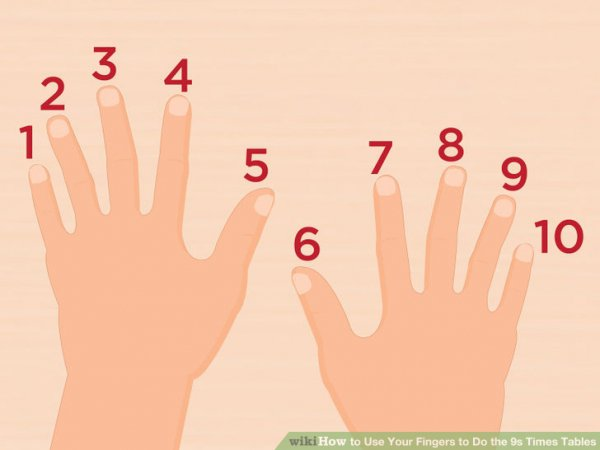You should've learned your multiplication tables back when you were in elementary school, but sometimes it'll slip your mind. If you have trouble multiplying nines, then all you have to do is use your fingers. If you're multiplying nine by five, put down your fifth finger and see what's left. Four fingers on the left hand and five on the right? That means the answer is forty-five.

## 2 Multiply Large Numbers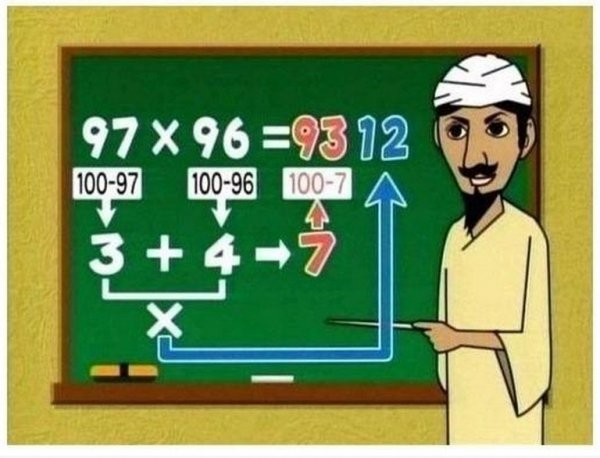If you don't have a calculator on you, and you don't have the patience to multiply a double digit number by another double digit number, then you can use this simple trick. Just subtract the numbers you have from 100, and add those two numbers together. The answer will start with the number you've gotten subtracted from 100, and will end with the two numbers multiplied together.

Related:Words used in the 1800s8 Ways to Look Polished Instantly ...

## 3 Pi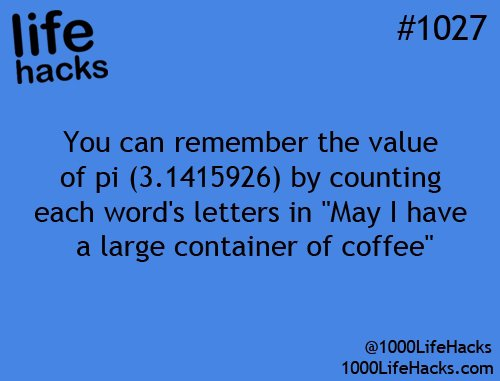You probably won't need to know much about pi, unless it's March 14th and your teacher wants to quiz you on how well you know its numbers. If that's the case, you shouldn't have too much of a problem. If you follow this little life hack, then you'll always remember the first eight digits of pi. Hey, that's more than most people remember.

## 4 Percentages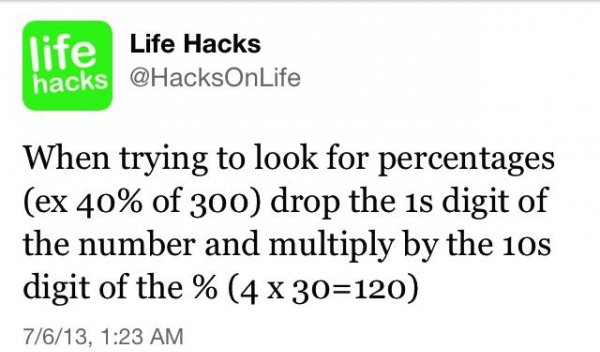Figuring out percentages is never any fun. That's why you should follow this advice. It'll help you figure out the answer more quickly than the traditional method that your teacher taught you.

## 5 Greater and Less than

You don't want to make a silly mistake on a test by getting your greater than and less than signs mixed up. That's why you should pretend that the symbol is an alligator. It always has its mouth facing toward the bigger number, because it wants to eat the biggest thing it can.

## 6 Which Day Falls on a Specific Date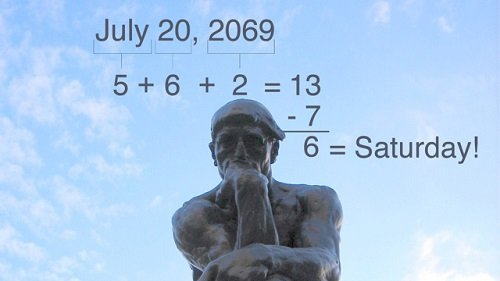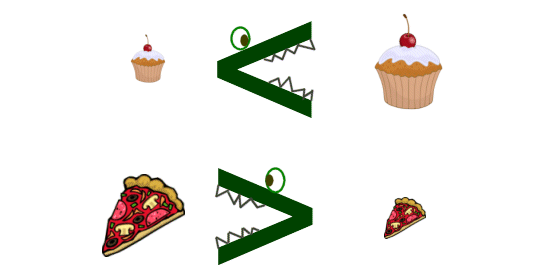You don't want to make a silly mistake on a test by getting your greater than and less than signs mixed up. That's why you should pretend that the symbol is an alligator. It always has its mouth facing toward the bigger number, because it wants to eat the biggest thing it can.

## 6 Which Day Falls on a Specific DateHere's a math hack that will actually come in handy in life. If you want to figure out what day your birthday will fall on next year, or if you can't remember what day your birthday fell on last year, you can use this trick. All it takes is a little bit of addition and subtraction. You can handle that!

## 7 Multiply 11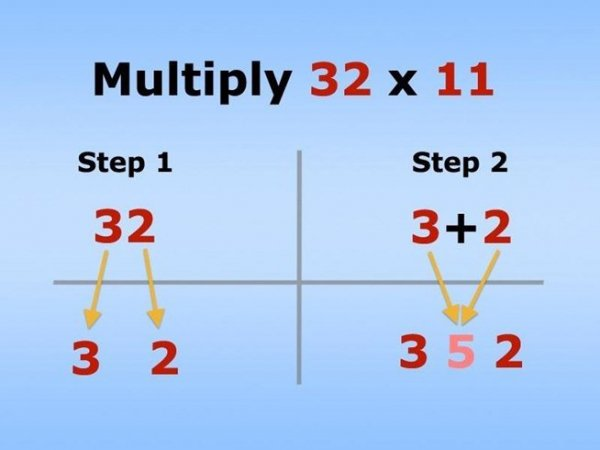If you have trouble multiplying double digit numbers by eleven, then this hack will help you out. All you have to do is add the two numbers together and put the solution in the center of those two numbers. Yes, it's that easy!

## 8 Convert FAHrEnhEit to CelSius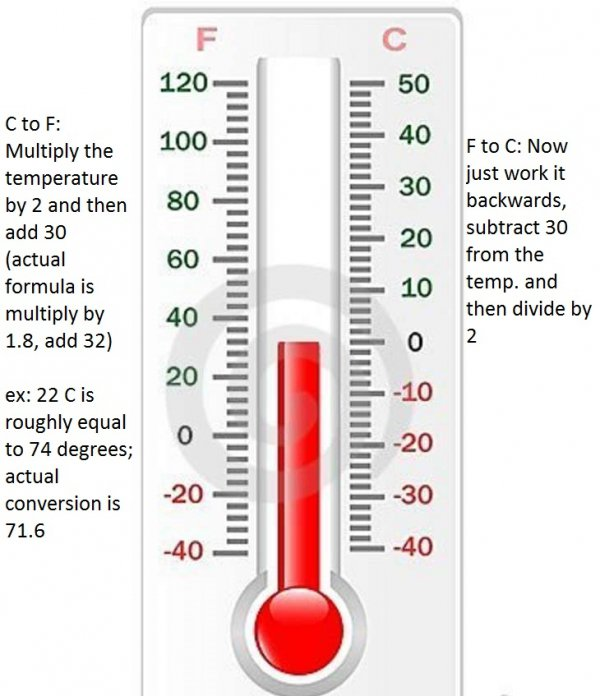Multiply the temperature by 2, then add 30. Yes, it truly is that simple! The actual formula is multiply by 1.8 then add 32 but to get a rough estimate this is much easier. 80 degrees Fahrenheit x 2=160+30=190 degrees Celsius.

## 9 Finding Fractions from Whole Numbers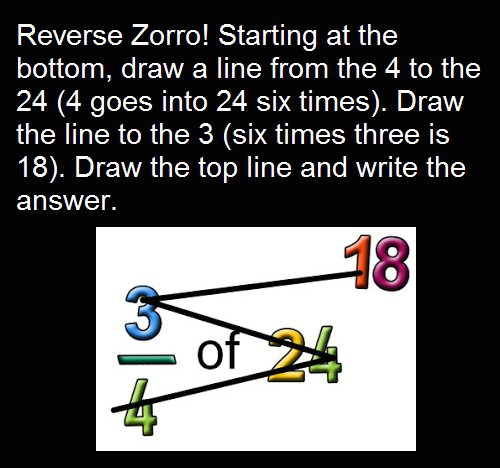This hack is called the reverse Zorro. Start at the bottom, draw a line from 4 to the 24. Divided this gives you 6. Then draw a line from the 24 to the 3, 6 x 3 is 18. Draw a line and your answer is written at the top.

## 10 Adding and SubTracting Fractions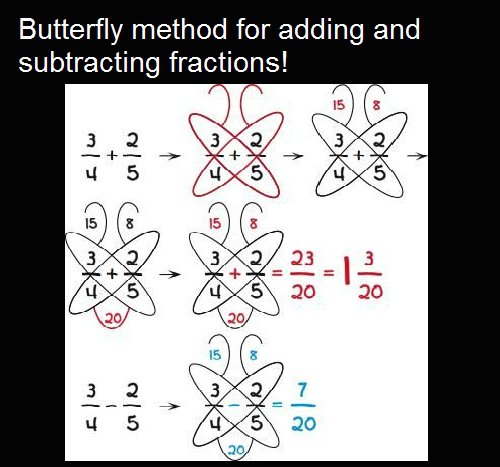This is super simple and seems almost too good to be true-but it is true! Use the butterfly method to add and subtract fractions. To add: Diagonally multiply numbers and put the answer in the antennas. 3 x 5=15 and 2 x 4=8. Then multiply the bottom two (4x5=20). Add the top two antenna numbers so your fraction is 23 over 20. Simplify. To subtract: Use the same basic method as addition until the end, subtract the antenna numbers and put over the multiplied. This gives you 7 over 20.

These math hacks might seem too simple to help you in your advanced courses, but they just might come in handy someday! What other math hacks do you know about?

Sources:
architecturendesign.net

• Tayyclayton14 Wait what?

• TiyAMSAG Where do those digits come from

• Lynzie01 Where did the 6 and 2 come from? I'm confused.

• Joxbrough I am confused by the method used to arrive at a specific day of the week...where do the digits 5,6 and 2 come from? Help!

• Reine I'm confused😂

• Pink The first one is my fave, been doing it since I was in elementary school!

• Tamara Some of these solutions are not working out. The first one does not work or the one that multiples by 11.

• Kay I'm still confused 😩 lol I hate math

• princessc7 3.14159265358979323846264338327950288419716939937510

Popular

Related

Recent